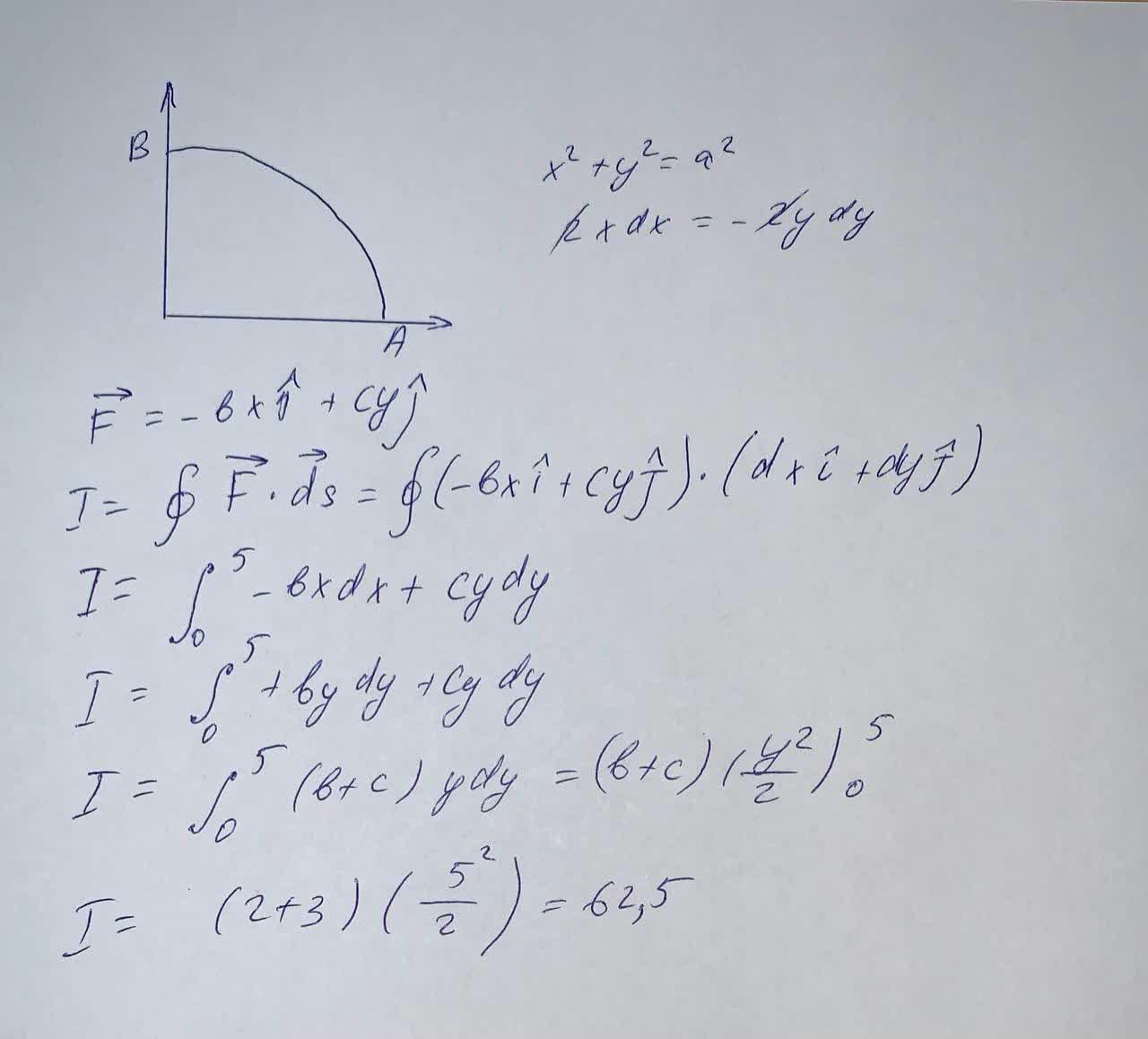Determine the line integral along the curve C from A to B. Find the parametric form of the curve C Use the vector field:\overrightarrow{F}=[-bx cy]Use the following values: a=5.b=2.c=3Anish Buchanan 2021-09-10 Answered

Determine the line integral along the curve C from A to B. Find the parametric form of the curve C
Use the vector field:
$$\overrightarrow{F}=[-bx\ cy]$$
Use the following values: $$a=5,\ b=2,\ c=3$$

• Questions are typically answered in as fast as 30 minutes

Solve your problem for the price of one coffee

• Math expert for every subject
• Pay only if we can solve itfalhiblesw

The line integral along the curve C from A to B is